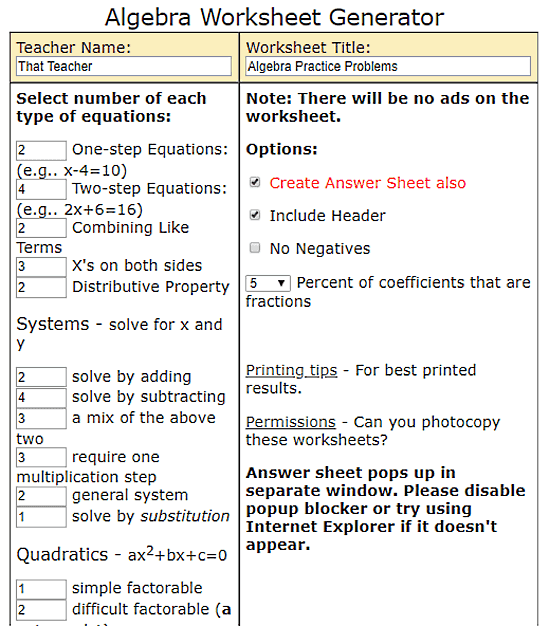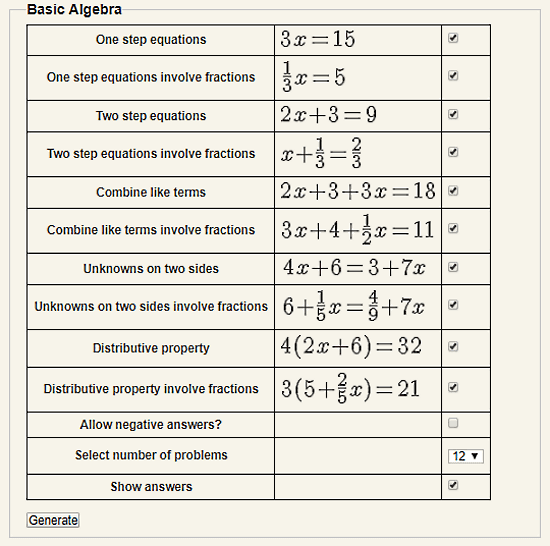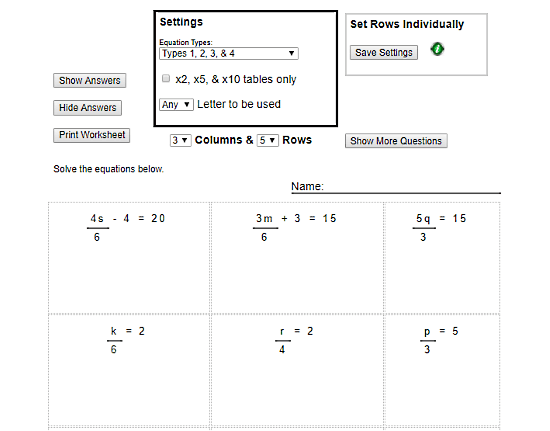Editor Ratings:
User Ratings:
[Total: 0 Average: 0]

In this article, I will cover 5 online algebra worksheet generator websites.

”Practice Makes A Man Perfect”. There is a fair chance that you might have heard this slogan at some point in your life. And, its true, you have to practice to be good at something. In terms of study, especially math, practice is most important. And, worksheets are a good medium to practice what you learn.

With the following 5 free websites, you can easily generate worksheets to practice algebra. These websites can be used by teachers, parents, and students to generate practice worksheets for algebra. Some of these websites let you cover all algebra topics in a single worksheet whereas other can only cover one or multiple (not all) topics. You can customize the worksheets as per your needs and generates printable worksheets with or without an answer key.

Let’s start the list and discuss these websites one by one.

Also, check out this Firefox Add-on To Solve Math Equations In Firefox Address Bar.

## Math.comMath.com is a free website that offers various types of study material for mathematics.  Here, teachers can plan their lessons, parents can access math resources for homeschooling and students can seek help in their homework.

Under the ‘Practice‘ section here, you can generate algebra worksheets. In the attached screenshot, you can see the interface of this algebra worksheet generator. It covers 15 types of algebra problems that include one-step equations, two-steps equations, systems of equations, quadratics equations and more. Simply enter the number of questions for the problem types which you want to include in your worksheet. If you don’t want to include any specific problem type, keep its number of questions to zero. You can also set the percent of coefficients for fractions. If you don’t want a problem that has a negative answer, you can skip that by checking the ‘No Negatives‘ options. And, if you want the answer sheets, check that option as well.

After selecting problem types and other options, enter teacher’s name and worksheet name at the top and click the ‘Generate‘ button. This will generate your worksheet which you can print or save as PDF with ‘Ctrl+P‘ option.

Try this Algebra Worksheet Generator here.

## WorksheetWorks.comWorksheetWorks.com is a free website where you can generate worksheets on various subjects. While generating a worksheet here, you can customize it as per your specifications. The algebra worksheet generator section shows you 17 algebra topics covering basic to advanced algebra topics. You have to select a topic on which you want to generate a worksheet. And, if you want to include all algebra topics in your worksheet, go with ‘Mixed Problem Types‘ option.

After selecting your algebra topic, you get options to customize your worksheets. Here, you can select a sub-topic for the problems like operation types, equation types, etc. depending on your topic. You can also adjust the visual appearance of your worksheet by changing the font, text size, number/value size (for problems), paper size and space between each problem. The number of problems is fixed for every topic means you can not generate worksheets with a specific number of problems.

When you generate your worksheet here, it gives you a downloadable PDF worksheet file. Along with the problems, the worksheet also includes the answer key in the end.

Here is the link to try this Algebra Worksheet Generator.

## MathMaster.orgMathMaster.org provides free educational resources for schools, parents, and students. These resources include videos and printable worksheets. Here, you can generate algebra worksheets for Basic algebra, Systems of equations, and Quadratic equation. You can only cover one of these topics in a single worksheet. When you select a topic, you get a list of sub-topics (or equations types). You can simply select the sub-topics that you want to include in your worksheet. After that, select the number of problems you want in the worksheet. You can select any number between 10 to 50. You also get options to allow negative answers and to include an answer key in the worksheet.

After configuring all the options, click the ‘Generate‘ button to generate your worksheet. Your worksheet will be open in a new tab, from there, you can print it or download it as PDF.

Give this Algebra Worksheet Generator a try here.

## HelpingWithMath.comHelpingWithMath is specially designed for parents who want to help their children with maths. It provides printable worksheets to help students practice what they learned. It has a simple algebra worksheet generator which parents/students can use to generate worksheets to practice algebra. Here, you can generate worksheets for four types of equations. You can use a single equation type, mix two equation types or mix all four equations types for the worksheet. You can set the number of questions by adjusting the number of rows and columns. It also gives you an option to use only x2, x5 &x10 tables for the fraction values. And, if you have some sort of problem with ‘x’, you can specify any other letter for your problems.

With ‘Show More Question‘ button, you can generate a new set of questions that will replace the current questions in the worksheet. You also have an option to include an answer key in your worksheet. The generated worksheet is a PDF file here which you can directly print or save to your computer.

Check out this Algebra Worksheet Generator here.

## SoftSchools.comSoftSchools provides free worksheets, games, quizzes, and other educational content on various subjects. With is Free Algebra Generator tool, you can easily generate algebra worksheets for basic and advanced algebra. To generate a worksheet, enter the title for the worksheets and set the number of problems by adjusting the rows and columns. Then, set a font and select an equation type. After that, click the ‘Generate‘ button to generate the worksheet. The generated worksheet will be open in a new tab which you can print to PDF using ‘Ctrl+P‘ command.

You can try this Algebra Worksheet Generator here.

### Wrap Up

These algebra worksheet generator websites are very simple to use. You can easily generate customized worksheet using any of them. Selecting one totally depends on your need as some of them can cover entire algebra in a single worksheet whereas others only cover sub-topics(s) under algebra.

 Editor Ratings: User Ratings:[Total: 0 Average: 0] Free/Paid: Free Tags: math equations